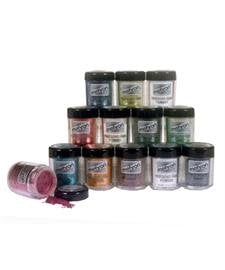# Mehron Shimmer Powders - Pearl Pr (0.17 Oz/4.8 Gm)

Vendor
Mehron
Regular price
\$31.49
Sale price
\$23.63
Quantity must be 1 or more

• E

• a

• c

• h

• 0

• .

• 1

• 7

• o

• u

• n

• c

• e

• s

• (

• 4

• .

• 8

• g

• m

• )

• c

• o

• n

• t

• a

• i

• n

• e

• r

• o

• f

• M

• e

• h

• r

• o

• n

• P

• e

• a

• r

• l

• C

• e

• l

• e

• b

• r

• e

• P

• r

• e

• c

• i

• o

• u

• s

• G

• e

• m

• P

• o

• w

• d

• e

• r

• w

• i

• l

• l

• a

• p

• p

• l

• y

• a

• b

• o

• u

• t

• 5

• 0

• e

• y

• e

• s

• h

• a

• d

• o

• w

• s

• o

• r

• o

• t

• h

• e

• r

• a

• c

• c

• e

• n

• t

• s

• .

• Color : Pearl

• Size : 0.17 oz/4.8 gm

Mehron Celebre Pearl Precious Gem Powders are highly pigmented shimmering powders that will add a jewel like luster to your face paint design. Rich, sparkling Gem Powders are made with a soft, silky powder that can be applied from head to toe for stage, dance, face painting and more. Precious Gem Powders are packaged in an easy-to-use shaker top container. 4.8 gram size.